Courses

# Test: Simple Mechanisms - 3

## 30 Questions MCQ Test Theory of Machines (TOM) | Test: Simple Mechanisms - 3

Description
This mock test of Test: Simple Mechanisms - 3 for Mechanical Engineering helps you for every Mechanical Engineering entrance exam. This contains 30 Multiple Choice Questions for Mechanical Engineering Test: Simple Mechanisms - 3 (mcq) to study with solutions a complete question bank. The solved questions answers in this Test: Simple Mechanisms - 3 quiz give you a good mix of easy questions and tough questions. Mechanical Engineering students definitely take this Test: Simple Mechanisms - 3 exercise for a better result in the exam. You can find other Test: Simple Mechanisms - 3 extra questions, long questions & short questions for Mechanical Engineering on EduRev as well by searching above.
QUESTION: 1

### In a Kinematic chain, a quarternary joint is equivalent to

Solution:

1 quarternary joint is equivalent to three primary joint and 1 turnery joint is equal to 2 binary joint.

QUESTION: 2

### The Kinematic planar chain shown in the given figure is a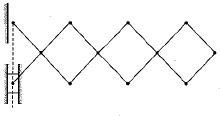Solution:

This device is known as telescopic hand.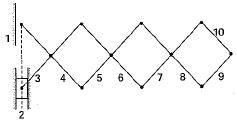It has 4 loop and 10 link hence degree of freedom is N - 9 i.e., one.

QUESTION: 3

### The number of degree of freedom of a planar linkage with 8 links and 9 simple revolute joint is

Solution: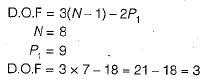QUESTION: 4

Which one of the following is a higher pair?

Solution:

In Belt and pulley due to line contact they formed higher pair.

QUESTION: 5

The method of obtaining different mechanism by fixing different links of a kinematic chain is known as

Solution:

Method of obtaining different mechanism by fixing different link of a kinematic chain is known as inversion.

QUESTION: 6

A four-bar chain has

Solution:

A four-bar chain consists of four revolute pair.

QUESTION: 7

The Grubler’s criteria for planar mechanism is given by f = 3(n-1) - 2j
j in the equation is

Solution:

In the equation
f = 3(n -1) - 2j
j = Number of lower pair

QUESTION: 8

Which of the following is not an inversion of simple slider crank mechanism

Solution:

Scotch yoke mechanism is an inversion of double slider crank mechanism.

QUESTION: 9

Quick return mechanism is used in

Solution:

Crank and.slotted lever type quick return mechanism is used in slotter machine.

QUESTION: 10

Which one of the following mechanism represents an inversion of the single slider crank chain

Solution:

Inversion of single slider crank mechanism are
1. Simple slider crank mechanism
2. Whitworth quick return mechanism
3. Crank and slotted lever mechanism
4. Hand pump mechanism

QUESTION: 11

For a 4-bar linkage in toggle position, the value of mechanical advantage is

Solution:

For toggle conditions mechanical advantage in infinite.

QUESTION: 12

Oldham’s coupling is the inversion of

Solution:

Inversion of double slider crank mechanism
1. Skotch Yoke
2. Elliptical Trammel
3. Oldham Coupling

QUESTION: 13

The mechanism used in a shaping machine is

Solution:
QUESTION: 14

The number of degrees of freedom of a five link planer mechanism with five revolute pairs as shown in the figure is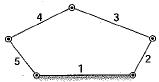Solution:

Degree of freedom
f = 3 (N - 1) - 2p1
where
p1 = number of revolute pair
Given, N = 5 , p1 = 5
Hence f = 3(5 - 1) - 2 x 5 = 2

QUESTION: 15

How many degree of freedom does the kinematic chain as shown in figure below have, when it undergoes planer motion.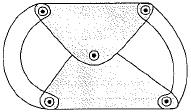Solution:

In this, N = 4
P1 = 5
DOF = 3(N - 1) - 2P1
DOF = 3(4 - 1) - 2 x 5
DOF = 9 - 10 = -1

QUESTION: 16

Which one of the following statements is correct?
Transmission angle is the angle between

Solution:

The angle between the output link and the coupler is known as transmission angle.QUESTION: 17

The length of the links of a 4-bar linkage with revolute pairs only are p, q, r and s units. Given that p < q < r< s. Which of these iinks should be the fixed one, for obtaining a ‘double crank’ mechanism?

Solution:
QUESTION: 18

In a four-bar linkage, the sum of the lengths of the smallest and the longest link is less than the sum of the lengths of the other two links. If the smallest link is fixed, the linkage becomes a

Solution:

For a four bar mechanism If S + L ≤ Sum of other two and if smallest link is fixed then crank-crank mechanism can be obtained.

QUESTION: 19

The input link, coupler link and the fixed link of a four bar mechanism have lengths 500 mm, 600 mm and 300 mm, respectively. When the input link is rotated by a motor, the output link can make complete revolutions if its length ‘L in mm satisfied the condition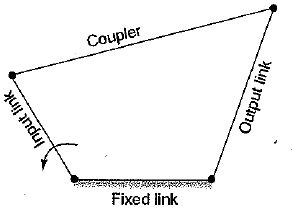Solution:

For complete revolution of output link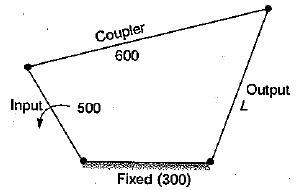If coupler is longest link, then
600 + 300 ≤ L + 500
L ≤ 400
If output is longest link, then
300 + L ≤ 600 + 500
L ≤ 800
Hence 400 < L < 800

QUESTION: 20

​Match the items in columns I and II.
Column-I
P. Higher kinematic pair
Q. Lower kinematic pair
R. Quick return mechanism
Column-ll
1. Grubler’s equation
2. Line contact
3. Euler's equation
4. Planer
5. Shaper
6. Surface contact

Solution:

P. Higher kinematic pair - Line contact
Q. Lower kinematic pair - Surface contact
R. Quick return mechanism - Shaper
S. Mobility of a linkage - Grubler’s equation

QUESTION: 21

Mobility of a statically indeterminate structure is

Solution:
QUESTION: 22

Which of the following statements, is incorrect?

Solution:
QUESTION: 23

The given figure shows below a/an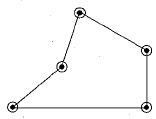Solution:Number of lower pair (Joint) = 5
Degree of freedom
f = 3(N - 1) - 2p1
f = 3(5 - 1) - 2 x 5
f= 2
Since DOF > 1
Hence it is unconstrained kinematic chain.

QUESTION: 24

A point on a connecting link of a double slider crank mechanism traces a

Solution:

It is known as elliptical trammel hance, it wiii trace elliptical path.

QUESTION: 25

The four bar mechanism shown in the figure (Given : OA = 3 cm, AB = 5 cm, BC = 6 cm, OC = 7 cm) is a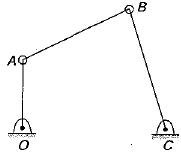Solution:

Shortest link OA = 3 cm
Longest link OC = 7 cm
Sum of shortest and longest link = 10 cm
Sum of other two = 5 + 6 = 11 cm
If sum of shortest and longest link is lesser than sum of other two then rocker-rocker mechanism is obtained.

QUESTION: 26

The double slider-crank chain is shown below in the diagram in its three possible inversions. The link shown hatched is the fixed link: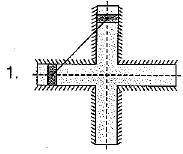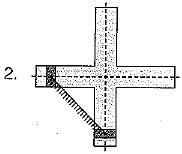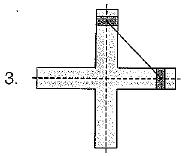Q. Which one of the following statements is correct?

Solution: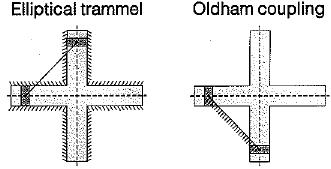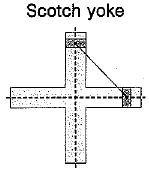QUESTION: 27

A planer mechanism has 8 links and 10 rotary joints. The number of degrees of freedom of the mechanism, using Grubler's criterion, is

Solution:

Degree of freedom is given by
f = 3(N - 1) - 2 p1 = 3(8 - 1) - (2 x 10) = 1

QUESTION: 28

For a mechanism shown below, the mechanical advantage for the given configuration is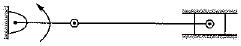Solution:

The configuration is a toggle condition and in toggle condition mechanical advantage is infinite.

QUESTION: 29

Match the following:
(Type of Mechanism)
P. Scott-Russel mechanism
Q. Geneva mechanism
R. Offset slider-crank mechanism
S. Scotch Yoke mechanism
(Motion Achieved)
1. Intermittent motion
2. Quick return motion
3. Simple harmonic motion
​4. Straight line motion

Solution:

P. Scott - Russel mechanism - straight line motion
Q. Geneva Mechanism - intermittent motion
R. Off - set slider crank mechanism - quick return motion
S. Scotch yoke mechanism - simple harmonic motion.

QUESTION: 30

Match List-I with List-lI and select the correct answer using the codes given below the lists:
List-I
A. Pantograph
B. Single slider crank chain
C. Double slider crank chain
D. Straight line motion
List-II
1. Scotch yoke mechanism
2. Double lever mechanism
3. Tchebicheff mechanism
4. Double crank mechanism
5. Hand pump
Codes:
A B C D
(a) 4 3 5 1
(b) 2 5 1 3
(c) 2 1 5 3
(d) 4 5 2 1

Solution: